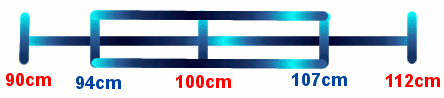# Boxplots

Before viewing this page, it would be helpful to learn how to calculate Quartiles.

Box-and-whisker plots are graphs showing five-number summary statistics (lowest score, 1st quartile, median or 2nd quartile, 3rd quartile, highest score) as follows:

• Lowest Score - the lowest score or number in the data
• 1st Quartile (also called Q1 or Lower Quartile) - the score below which lies one quarter of the data
• Median (also called Q2 or 2nd Quartile) - the score below which lies one half of the data
• 3rd Quartile (also called Q3 or Upper Quartile) - the score below which lies three quarters of the data
• Highest Score - the highest score or number in the data

## Did You Know That...?

People from the Netherlands (Dutch people) are the world's tallest people with an average height of 1.85 metres. As a nation, people from Japan are the world's shortest people with an average height of 1.63 metres.

## Example - Boxplot of Dutch Jeans' Sizes

The waist to ankle lengths (in cm) for 15 Dutch men are listed below. By first calculating the five-number summary, show this information as a boxplot.

Ordered Data (from smallest to largest) - 90, 91, 92, 94, 97, 98, 98, 100, 100, 105, 106, 107, 110, 111, 112

n = 15
Lowest Score = 90 cm

 1st Quartile = (n + 1) ÷ 4 = (15 + 1) ÷ 4 = 4th man = 94 cm Median = (n + 1) ÷ 2 = (15 + 1) ÷ 2 = 8th man = 100 cm 3rd Quartile = (n + 1) ÷ 4 × 3 = (15 + 1) ÷ 4 × 3 = 12th man = 107 cm

Highest Score = 112 cm## Question - Boxplot of Japanese Jeans' Sizes

The waist to ankle lengths for 11 Japanese men are listed below. By first calculating the five-number summary, show this information as a boxplot.

Ordered Data (in cm) - 70, 71, 73, 75, 75, 78, 79, 82, 85, 86, 88